Search

Using Identities to EvaluateOnce you've learned some trig identities, you can use them to evaluate angles exactly. Yes, you can always get a decimal approximation with your calculator, but it's good to learn the logic of using the identities, so you can exercise your skills at pattern-finding and -matching.

• Evaluate cos(75°) exactly.
• I know the exact values for the cosine of 30° and 45°, and 75° = 30° + 45°, so I'll use the angle-sum identity to compute this value "exactly":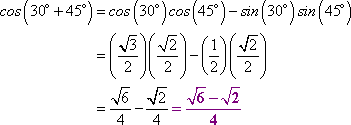You can check your answers to this sort of exercise by plugging each of the trig expression and the "exact" expression into your calculator. If the displayed values are the same (0.2588190451, in this case), then you know you've done the evaluation correctly.

• Evaluate tan(15°) exactly.
• Since 15 = 45  30, I'll use the angle-difference identity for tangent: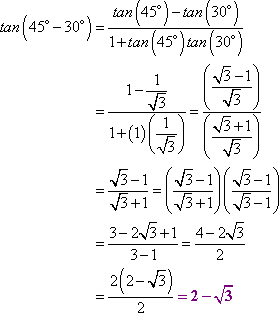If your book prefers radians, you'll be asked to evaluate expressions like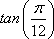, which is the same angle as above. The sums and differences can be a bit harder to recognize in radian form: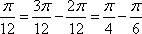A couple of useful sums are: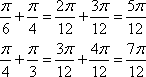If you work better with degrees, then convert radian-measure angles to degrees, find the sums and differences, and convert back to radians.

• Evaluate sin(120°) using a formula.
• Since 120° is twice 60°, I'll use the double-angle formula for sine:

sin(2×60°) = 2sin(60°)cos(60°)

Since 60° is a basic reference angle, I can now finish the evaluation by plugging in the values I've memorized: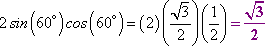• Since 22.5 is half of 45, I'll use the half-angle identity for cosine: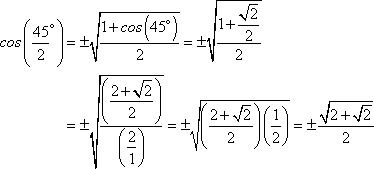Since 22.5° is in the first quadrant, then the cosine value is positive, so I'll take the positive root for my answer: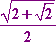• Find cos(2x) if sin(x) = 5/13 and x is in the third quadrant.
• From the sign on the sine, I could only tell that x was in QIII or QIV; that's why they had to specify the quadrant for x. By the Pythagorean Theorem, I can find the third side of the triangle from the sine's ratio value:

So the adjacent side has a length of ±12. Since the angle x is in the third quadrant, then the "length" is 12. Plugging into the double-angle formula, I get: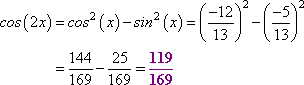In this case, I didn't really "need" the quadrant information; the squaring and subtraction took care of the signs. But sometimes you will need to pick one of two values, as in the previous example. Don't get lazy with the "plus / minus" signs. Keep track of where you are and where you're going, and pick the appropriate sign, as needed.

 Cite this article as: Stapel, Elizabeth. "Using Identities to Evaluate." Purplemath. Available from     https://www.purplemath.com/modules/ideneval.htm. Accessed [Date] [Month] 2016

Study Skills Survey

Tutoring from Purplemath
Find a local math tutor